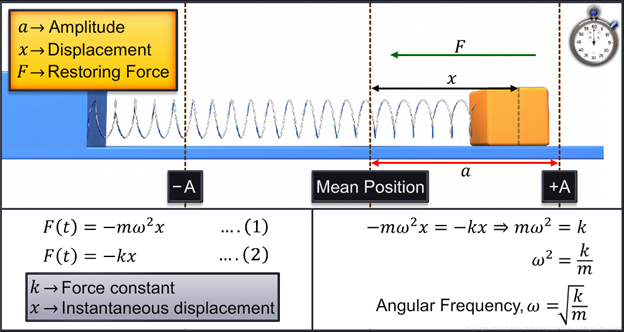Notes On Force Law in SHM - CBSE Class 11 Physics
Consider a body that executes simple harmonic motion with amplitude ‘a’. In this case, the force,always acts towards the mean position of the body and is proportional to the displacement of the body. Hence, this force is called the restoring force in simple harmonic motion. For simplicity, if we assume the initial phase Ф to be zero, then the displacement of the body isAccording to Newton’s second lawLet this be Equation 1.Hence, angular frequency is equated as shown in the above picture. Thus, if the force is directly proportional to displacement, it is called a linear harmonic oscillator. On the other hand, if the force depends on displacement, but is not directly proportional to displacement, it is called a non-linear harmonic oscillator. You have now reached the end of this module. In this module, you learned that:   A linear harmonic oscillator is one where the force is directly proportional to displacement.   A non-linear harmonic oscillator is one where the force is dependent on displacement but not exactly directly proportional.

#### Summary

Consider a body that executes simple harmonic motion with amplitude ‘a’. In this case, the force,always acts towards the mean position of the body and is proportional to the displacement of the body. Hence, this force is called the restoring force in simple harmonic motion. For simplicity, if we assume the initial phase Ф to be zero, then the displacement of the body isAccording to Newton’s second lawLet this be Equation 1.Hence, angular frequency is equated as shown in the above picture. Thus, if the force is directly proportional to displacement, it is called a linear harmonic oscillator. On the other hand, if the force depends on displacement, but is not directly proportional to displacement, it is called a non-linear harmonic oscillator. You have now reached the end of this module. In this module, you learned that:   A linear harmonic oscillator is one where the force is directly proportional to displacement.   A non-linear harmonic oscillator is one where the force is dependent on displacement but not exactly directly proportional.

Previous
Next